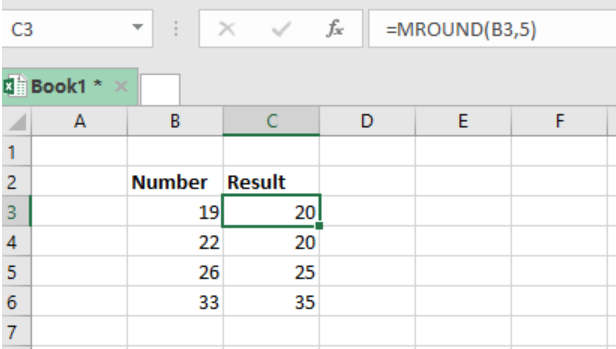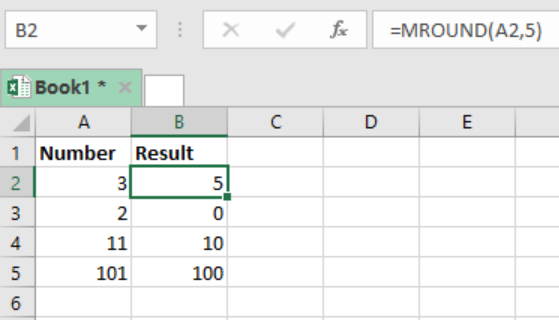Get instant live expert help with Excel or Google Sheets“My Excelchat expert helped me in less than 20 minutes, saving me what would have been 5 hours of work!”

#### Post your problem and you'll get expert help in seconds

Your message must be at least 40 characters
Our professional experts are available now. Your privacy is guaranteed.

# How to Round to Nearest 5 in Google Sheets and Excel

When working with Google sheets and Excel sheets, we may be required to round to nearest 5 or its multiple. In this post, we shall provide a clear illustration on how to round to nearest 5 in Google sheets and Excel using the MROUND function.Figure 1: How to perform excel roundup and excel round down to nearest 5

## General syntax of the formula

`=MROUND(number,5)`

For us to know how to round to nearest 5 in Google sheets and Excel, we must first be familiar with the MROUND function. This function is fundamental when it comes to rounding numbers to multiples of 5.

## Understanding MROUND function in rounding to nearest 5

To better understand how to round to nearest 5 in Google sheets and Excel, we have to look at the example above; In the example, we have the formula in cell C3 as;

`=MROUND(B3,5)`

Note that B3 refers to the number in the sheet. We will realize that the number in cell B3 is 19, but we have the result as 20. This is because 20 is the nearest multiple of 5 from 19. This is so that the function performs an excel roundup to the next figure, which is 20.

## ExampleFigure 2: How to perform excel round to nearest integer

To further explain how to round to nearest 5 in Google sheets and Excel, let us look at example 1 above.

In this example, we have our numbers in column A and the results in column B.

We have put our formula, `=MROUND(A2,5)`

In cell A2 where we have 3, it will be rounded up to 5, given that it is the nearest multiple of 5 from 3. When we consider cell A3 where we have number 2, the function will perform an excel round down to get 0. This is because 0 is closer to 2 than to 5.

## Conclusion

Learning how to round numbers in Excel is valuable in many instances. The knowledge can be utilized in many areas, such as financial sectors and others. There are various tutorials that can help one get the necessary excel round skills that can prove fundamental during various tasks.

Most of the time, the problem you will need to solve will be more complex than a simple application of a formula or function. If you want to save hours of research and frustration, try our live Excelchat service! Our Excel Experts are available 24/7 to answer any Excel question you may have. We guarantee a connection within 30 seconds and a customized solution within 20 minutes.

### Did this post not answer your question? Get a solution from connecting with the expert.Another blog reader asked this question today on Excelchat:## Subscribe to Excelchat.coAnother blog reader asked this question today on Excelchat: